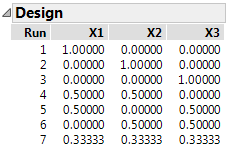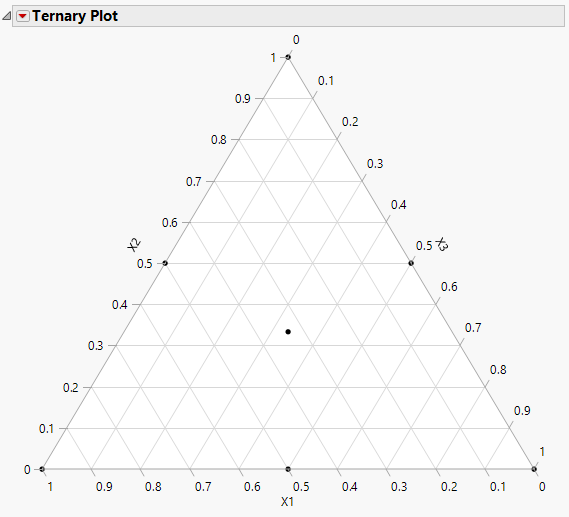Publication date: 05/24/2021

## Simplex Centroid Design

A simplex centroid design of degree k with n factors consists of mixtures with the following characteristics:

all one factor mixtures or pure blends

all blends of two factors at equal levels

additional blends, up to k factors at a time blended at k equal levels

A center point run with equal amounts of all the ingredients is included when k is equal to the number of factors.

Note: This design can be used with lower bounds on one or more factors.

### Creating the Design

To create an example simplex centroid design:

1. Select DOE > Classical > Mixture Design.

2. Use three factors for this example. No changes need to be made to the Factors section.

3. Click Continue.

4. Click Simplex Centroid to generate the design

Note: The default setting of k = 2 was used. There is a text box that enables you to adjust the value of k.

Figure 13.8 Three Factor Simplex Centroid Design of Degree 2.5. Click Make Table.

#### Visualize the Design

6. From design table, select Graph>Ternary Plot.

7. Select X1, X2, and X3 and click X, Plotting, and then click OK.

Figure 13.9 Ternary Plot for Simplex Centroid Design